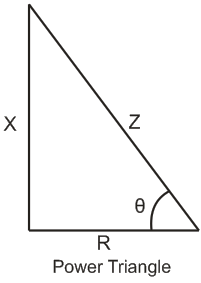# MCQs on Electric Circuits

##### Page 60 of 63. Go to page 1 2 3 4 5 6 7 8 9 10 11 12 13 14 15 16 17 18 19 20 21 22 23 24 25 26 27 28 29 30 31 32 33 34 35 36 37 38 39 40 41 42 43 44 45 46 47 48 49 50 51 52 53 54 55 56 57 58 59 60 61 62 63
01․ In which braking back emf exceeds supply voltage?
Regenerative.
Dynamic.
Plugging.
None of these.

When back emf is greater than supply voltage machine acts as a generator. This phenomenon is called regeneration and this form of braking is called regenerative braking.

02․ Third pin of a 3 pin plug is thicker and longer
for designing purpose.
for protection purpose.
all pins are of same size.
none of these.

Third pin is for earth. In case of any earth fault heavy current flows through the thicker pin without over heating or burning the contacts. Thicker earth pins provide more surface area.

03․ What is the reactive power in AC operation?
Real power × sinθ.
Apparent power × sinθ.
Real power × cosθ.
Apparent power × cosθ.

The below figure represents the power triangle. The perpendicular (X) represents the reactive power (or reactive impedance), base (R) represents active power (or resistance) and hypotenuse (Z) represents apparent power (or impedance).04․ Power factor can be defined as
both option B and C.
cosine of angle between voltage and current.
ratio of resistance versus impedance.
sine of voltage and current.

Power factor is defined as the ratio of active power to apparent power. Active power is I*R or VIcosθ. Apparent power is I*Z or VI.

05․ A 60 Hz frequency voltage would cause a bulb to turn on and off
60 times per second.
120 times per second.
70 times per second.
80 times per second.

The bulb will be turned on 60 times and turn off 60 times in one second.

06․ Our household apparatus are connected in
parallel.
series.
series and parallel combination.

The household apparatuses are connected in parallel so that equal voltage is applied across them as they operate on a rated voltage.

07․ Total instantaneous power supplied by a 3 - φ AC supply to a balanced RL load is
zero.
constant.
pulsating with zero average.
pulsating with non zero average.

Total instantaneous power supplied by a 3 – φ AC supply to a balanced RL or RC load is always constant.

08․ What are the points to be mentioned in specifying main switches?
Current rating.
Both A and B.
None of these.

Voltage grade, current rating, no. of poles, types of enclosures, types and position of fuses are the points to be mentioned in specifying main switches. Ex - 500 Volt grade, 100 Amp, triple pole and neutral , iron clad switch fuse.

09․ In direct arc furnace which of following is of high value?
Voltage.
Current.
Power factor.
All of above.

Since the arc is formed between the electrodes and charge, heat is also produced by flow of current through the change which offers very low resistance. Hence we can say taht current is of high value.

10․ Efficiency of electrical machines should be calculated by measuring
output and input.
losses and input.
losses.
losses and output.

We know that the input power of any machine is the summation of losses and the output power and also the efficiency is the ratio of the output power to the input power. Hence, losses are mainly concentrated to calculate the efficiency of electrical machines.

<<<5859606162>>>